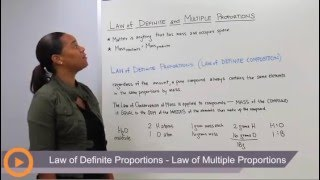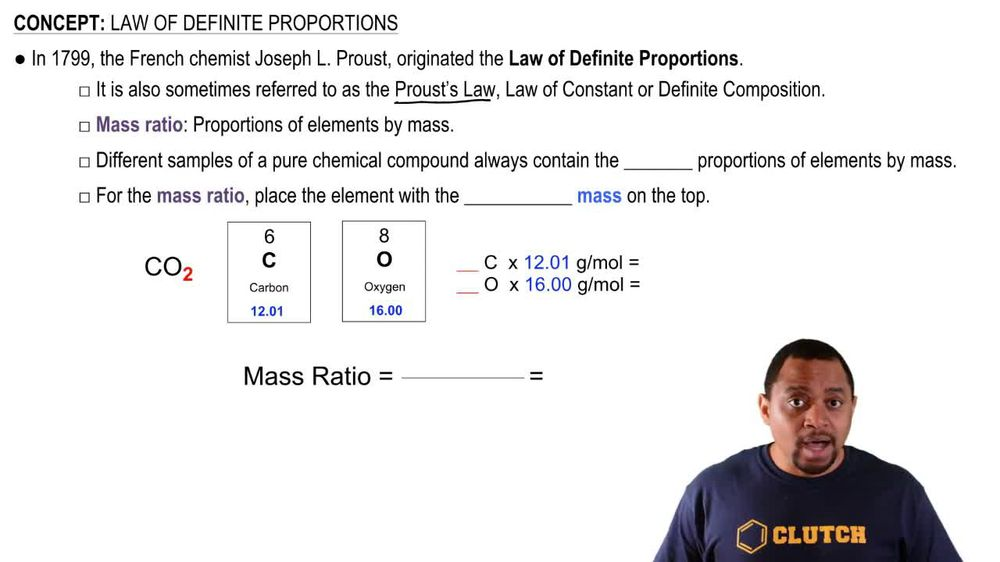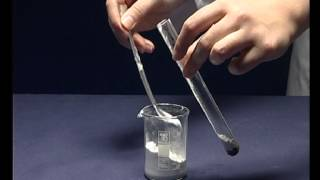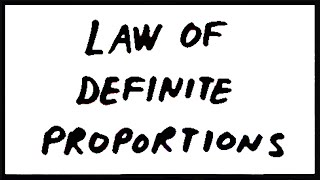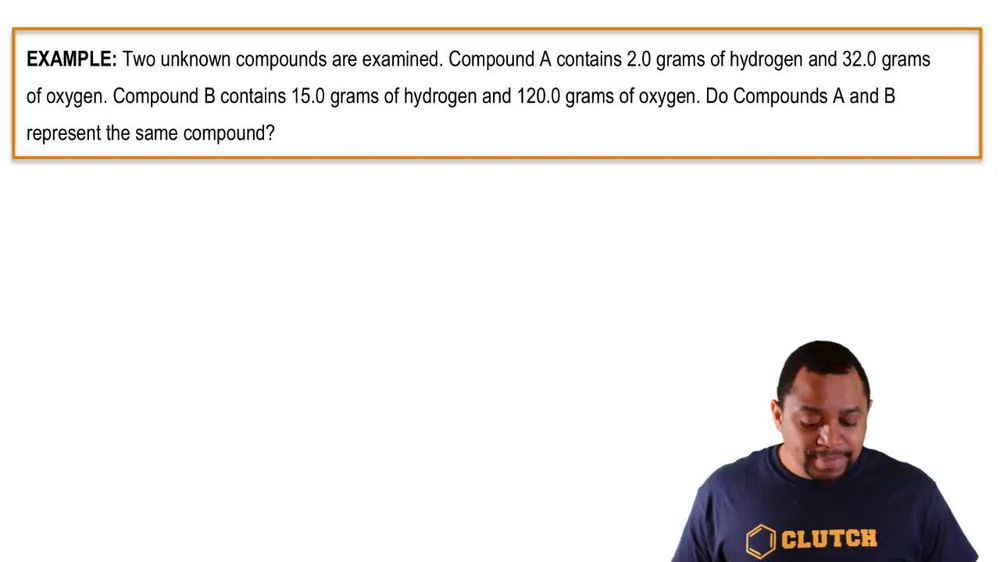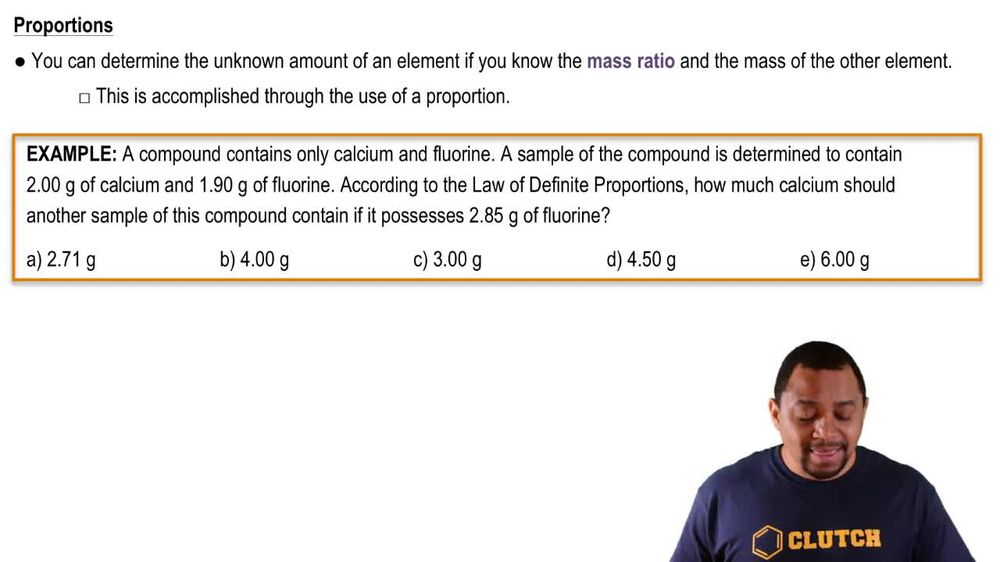Start typing, then use the up and down arrows to select an option from the list.
1. 2. Atoms & Elements2. Law of Definite Proportions
Problem

# The mass ratio of sodium to fluorine in sodium fluoride is 1.21:1. A sample of sodium fluoride produces 28.8 g of sodium upon decomposition. How much fluorine (in grams) forms?

Relevant Solution1m
Play a video:
Hello everyone today. We are being given the following problem and asking to solve for it, cesium iodide has a cesium to iodine mass ratio of 1.4721. When a sample of the cesium iodide is completely decomposed, 27.88 g of cesium is produced. What mass of iodine in grams is produced. So first we want to make note of our cesium iodine ratio And we're gonna use this ratio to calculate for the mass of iodine in this sample. So our massive iodine is 27. g of cesium. Everybody multiplied by that ratio that we have. So for every one g of iodine that we have, we have 1.47 g of cesium. And this is per that ratio given to us in the question stem Our units are going to cancel out our g of season, cancel out. And we will be left with 26.63 g of iodine as our final answer. I hope this helped. And until next time